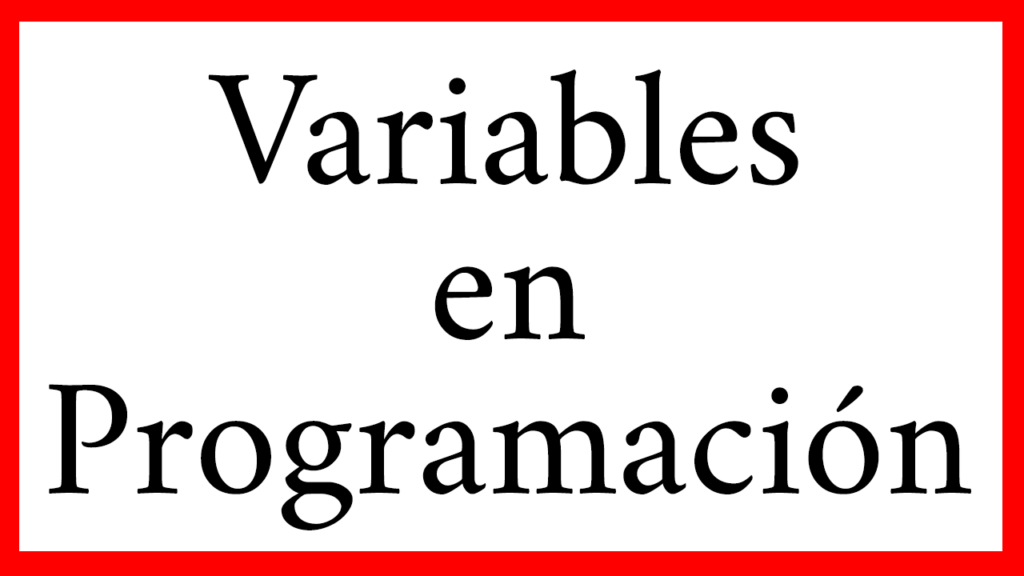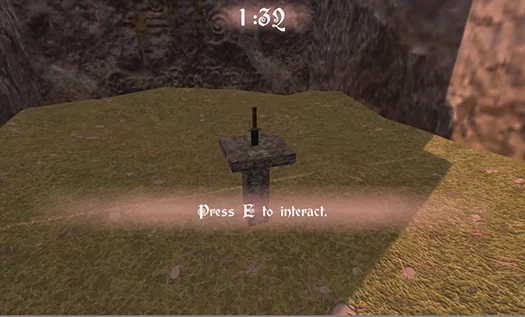# Variables in Programming

## Introduction

In this article we are going to explain what is a variable in programming, what types of variables there are, what they are used for and show some examples of variables in C#, in the development of video games with Unity.

The following video summarizes some of the information in this article. You can watch it before you start to get a general idea.

### What is a Variable in programming?

Variables are used in many disciplines, perhaps the most common example is the variable x in mathematics, which is generally an unknown value that we must find. In programming variables are a somewhat different concept.

To understand the essence of computer variables we need to know a little about computer memories.

Memories store information in the form of bit registers (i.e. sets of values that can be 0 or 1). This information will be interpreted and used by our programs.

Memories in turn have many of these records, so each record has an address that can be found, read, and written.

Then we can say that a variable is a DATA that is stored in the memory of the computer.

The identification name we assign to it is associated with the address inside the memory and the value of the information it contains.

### Examples of variables in programming

The variables in programming can be of different type, the election of the type will depend on the information that we want to store in it.

In figure 1 we see different types of variables and their assigned value.

The variable “age” contains the value 22, this means that when we use the word “age” in our program, indirectly we will be using the value 22.

If we do the operation “2*age” (2 multiplied by age) it will have a result of 44.

### Choosing Names for Variables

Figure 2 shows variables that have been defined with confusing names. This happens very often when we want to solve things fast.

It is important to choose representative names of the variables, because after a while we forget what we did and when we have to make changes it is difficult to understand.

The variable “house” in figure 2 has the value “false”. This variable could have been used, for example, to know if a character is in his house and if it is true to restore his health.

When putting a context it makes sense that “house” is false, this means that the character is not at home. However, it may take us a while to figure out what the function of the variable was.

We can save the effort by simply defining the variable as for example “characterAtHome”. Now the name of the variable tells us its function!

### Types of Variables in Programming

Let’s know the most basic variables that we are going to use to solve our problems. The examples are taken from the Labyrinth Game Series (click on the link to go to the main page of the series).

#### Variables type Boolean (bool)

The bool variables are named after the Boole Algebra developed by mathematician George Boole. They can be found in 2 possible states: True or False. We use them to solve logical operations.

In the method seen in figure 3, we define the bool “validSelection” and initialize it with the false value.

Inside the while loop you select a random piece from the labyrinth and check if it is far enough from the character.

If the labyrinth piece is very close to the character, validSelection remains false and the program chooses another labyrinth piece again.

If the labyrinth piece is far enough away, validSelection will become true and the program will go ahead using that piece.Fig. 4: The object to be found in the labyrinth is a pedestal with a sword.

#### Integer type variables (int)

The “int” type variables do not serve to store positive and negative integer values.

In the method shown in figure 4, you see several integers, “nPieces”, “labyrinthPieces.Length”, “nClocks”, the numbers 0 and 1 and the iteration variable “i”.

Let’s take the case of the iterator variable “i”, it is declared and initialized with value 0 inside the for loop and it is used to execute the “placeAClock” method as many times as the value of nClocks is.

For example if we want to have 10 clocks in the scenario, we make “nClocks” worth 10, then the for loop will execute 10 times the “placeAClock” method.

#### Float Variables

Floating point variables are used to represent real numbers. That is to say, besides positive and negative integers we can represent numbers with decimal part. This type of variables can serve us for example to represent physical magnitudes.

In the video of the Halloween 2018 special we defined a script for the NPC Lucy, a specter that dwells in the labyrinth. Lucy chases the character with some speed.

To solve this behavior we make a linear interpolation between the position of the character and Lucy’s position using as parameter the float “velocity” multiplied by the float “Time.deltaTime”.

#### String Variables

Strings are not a variable in itself, but instances of a class, however many development environments allow us to treat strings as primitive variables.

We use them to store text.